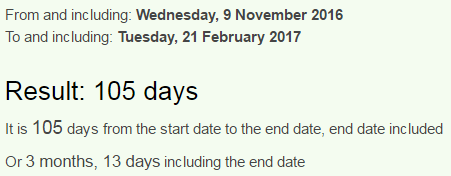CipherFeb 21, 2017

"McCain steps up Trump criticism" - Here is why this is coming today 221/33

John McCain appeared on CNN  to "criticize" Trump today, and it's very interesting how they planned this event (CNN's - McCain Steps up Trump Criticism)
Trump criticism = 7+9+6+14+11 + 24+9+18+7+18+24+18+8+14 = 187 (Reverse Ordinal)
Lately there was a lot of 187 coding coming up in the news in relation to Trump, 187 is a very relevant number and a homicide code apart from that, the numbers that appear in this story could be interpreted to be assassination codes, however I will ignore that narrative completely, in my previous post I talked about 187 connections and other things relating to thatIn this video he actually mentions how the New World Order needs media to stay "FREE" in order to maintain this incredible system of "democracy" they established. He says: "Fundamental part of the New World Order is free press". Afterwards he even emphasizes how media being "FREE" is extremely important to him... What a joke.. These spineless morons truly have no shame or consciousness of any kind8/29/1936 - 8+2+9 + 1+9+3+6 = 38 - McCain = 5+6+6+8+9+4 = 38 (Reverse Reduced)
He's supposedly born in "Coco Solo" = 6+3+6+3 + 8+3+6+3 = 38 (Reverse Reduced)
"Panama Canal Zone" = 16+1+14+1+13+1 + 3+1+14+1+12 + 26+15+14+5 = 137 (Ordinal) - 33rd prime"Donald" = 4+50+40+1+20+4 = 119 (Jewish)
"Mr Trump" = 13+18 + 20+18+21+13+16 = 119 (Ordinal)
There's more connections that can be made to Trump from his name though
"John Sidney McCain III" = 10+15+8+14 + 19+9+4+14+5+25 + 13+3+3+1+9+14 + 9+9+9 = 193 (Ordinal)
44th prime number is 193 - Donald Trump is the 44th person to be the president but he is 45th term president also connecting to "John McCain"
John McCain = 1+6+8+5 + 4+3+3+1+9+5 = 45 (Reduced)

The length of the video is 2:57 (177 seconds) - and of course this moron is showing up here 177 days after his birthdayHere is how they measured to see when they want to have him show up to "criticize" Trump, they measured from Inauguration day to his birthday in 2017, which is 221 days a lot like this date 2/21/2017Also you can see how this date is coming 31 weeks and 4 days before his birthday a lot like Pi - 3.14, which is very relevant to Trump and a lot of the coding from the last few months, Trump won the election vote on 314th day of the year (11/9) and now here we have McCain(119) criticizing Trump on a day that leaves 314 days in the leap years"Pi" = 16+9 = 25 (Ordinal) - Super Bowl 51 took place on 2/5 - 2 months and 27 days after Trump won the election voteBut 2017 isn't a leap year, and there is also a connection to 313 of course, if we measure from 11/9/2016 to 2/21/2017, we see 3 months and 13 daysNow we also see 105 days (Masonry = 105, Zionism = 105), which can be seen as 15 which connects to date numerology of 2/21/2017
2/21/2017 - 2+2+1+2+0+1+7 = 15 (47 is 15th prime number - President = 47) - "Trump" = 7+9+6+14+11 = 47 (Reverse Ordinal)

So they got 221 days by measuring from Inauguration to McCain's birthday and then they measured to 2/21 from Inauguration dayNote that this story came on a date numerology of 33 (2+21+2+0+1+7 = 33), 33 days after Inauguration day that had date numerology of 38 (1+20+17 = 38 - McCain = 38)
"John McCain" = 10+15+8+14 + 13+3+3+1+9+14 = 90 (Ordinal)
"Ninety" = 5+9+5+5+2+7 = 33 (Reduced)"Sen. John McCain" = 8+22+13 + 17+12+19+13 + 14+24+24+26+18+13 = 223 (Reverse Ordinal)
"The Arizona Republican" = 7+19+22 + 26+9+18+1+12+13+26 + 9+22+11+6+25+15+18+24+26+13 = 322 (Reverse Ordinal)
The Synagogue of Satan = 223, and 322 is the number of Skull & Bones...